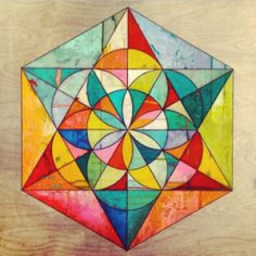# CCSS Gr. 7 Geometry

Topic:
Geometry
========UNDER CONSTRUCTION======== Common Core Standards for Grade 7 – Geometry Draw construct, and describe geometrical figures and describe the relationships between them.
• CCSS.Math.Content.7.G.A.1 Solve problems involving scale drawings of geometric figures, including computing actual lengths and areas from a scale drawing and reproducing a scale drawing at a different scale.
• CCSS.Math.Content.7.G.A.2 Draw (freehand, with ruler and protractor, and with technology) geometric shapes with given conditions. Focus on constructing triangles from three measures of angles or sides, noticing when the conditions determine a unique triangle, more than one triangle, or no triangle.
• CCSS.Math.Content.7.G.A.3 Describe the two-dimensional figures that result from slicing three-dimensional figures, as in plane sections of right rectangular prisms and right rectangular pyramids.
Solve real-life and mathematical problems involving angle measure, area, surface area, and volume.
• CCSS.Math.Content.7.G.B.4 Know the formulas for the area and circumference of a circle and use them to solve problems; give an informal derivation of the relationship between the circumference and area of a circle.
• CCSS.Math.Content.7.G.B.5 Use facts about supplementary, complementary, vertical, and adjacent angles in a multi-step problem to write and solve simple equations for an unknown angle in a figure.
• CCSS.Math.Content.7.G.B.6 Solve real-world and mathematical problems involving area, volume and surface area of two- and three-dimensional objects composed of triangles, quadrilaterals, polygons, cubes, and right prisms.